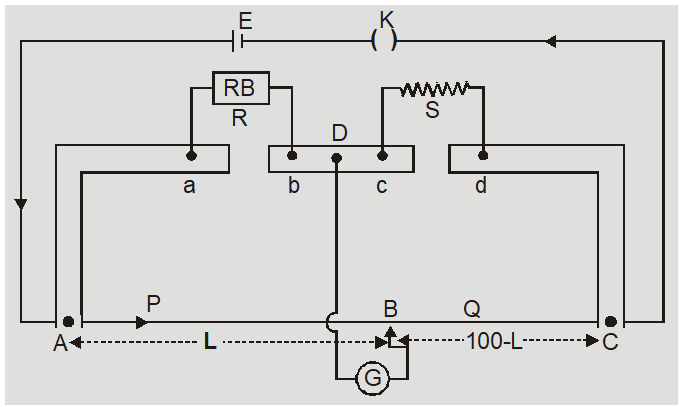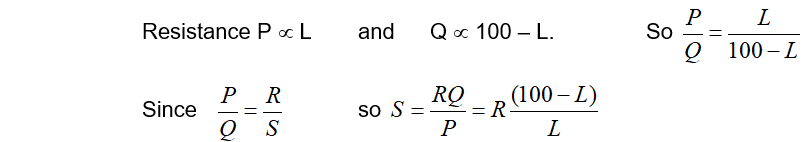Download the eSaral app and start learning from Kota's top IITians and doctors.

# Wheatstone Meter Bridge - Circuit Diagram || Current Electricity Class 12, JEE NEET

A Meter Bridge also called a slide wire bridge is an instrument that works on the principle of a Wheatstone bridge. A Wheatstone meter bridge is used in finding the unknown resistance of a conductor as that of in a Wheatstone bridge.

The wire is made of manganin  because they have low temperature coefficient and large resistivity. The wires are soldered to two thick L shaped copper strips with binding terminals. Between these there is a third copper strip. There are two fixed gaps ab and cd.(1) It is used to measure unknown resistances $(1 \Omega \text { to } 1000 \Omega)$, for comparision of two unknown resistances and specific resistance of material of wire. (2) For better accuracy R must be adjusted to obtain L between 40 to 60 cm. It has maximum accuracy when $\mathrm{L} \approx 50 \mathrm{cm}$. (3) It is more sensitive than PO box but suffers from end resistances.

### SOLVED EXAMPLES

Ex. An unknown resistance S is placed on the left gap and known resistance of $60 \Omega$ in right gap of meter bridge. The point is obtained at 40 cm from left end of bridge. Find the unknown resistance? Sol. $S=R \frac{(100-L)}{L}=60 \frac{(100-60)}{60}=40 \Omega$
Ex. In a meterbridge the length of wire is 100 cm. At what point is balance point obtained if two resistances are in ratio 2 : 3? Sol. $\frac{P}{Q}=\frac{L}{100-L}=\frac{2}{3} \quad$ so $\quad 3 \mathrm{L}=200-2 \mathrm{L} \quad$ or $\quad L=\frac{200}{5}=40 \mathrm{cm}$   Also Read: